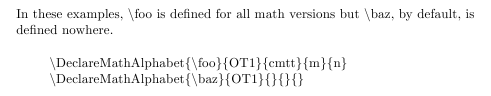## 網頁

### 可以讓你省時省力的技巧– \verbIn these examples, \foo is defined for all math versions but \baz, by default, is defined nowhere.

In these examples, \textbackslash{foo} is defined for all math versions but \textbackslash{baz}, by default, is defined nowhere.

\DeclareMathAlphabet{\foo}{OT1}{cmtt}{m}{n}}
\DeclareMathAlphabet{\baz}{OT1}{}{}{}}

\textbackslash{DeclareMathAlphabet\{\textbackslash{foo}\}\{OT1\}\{cmtt\}\{m\}\{n\}}\\
\textbackslash{DeclareMathAlphabet\{\textbackslash{baz}\}\{OT1\}\{\}\{\}\{\}}

1. 在源文件 (*.tex) 的前言區 (Preamble)，加入下列指令。
\usepackage{shortvrb}    % 用于大量使用 LaTeX 特殊意義符號的情形
\MakeShortVerb|
2. 在需要的地方 ，使用 | 抄錄文字 | 語法。
例如：| \DeclareMathAlphabet{\foo}{OT1}{cmtt}{m}{n}} |